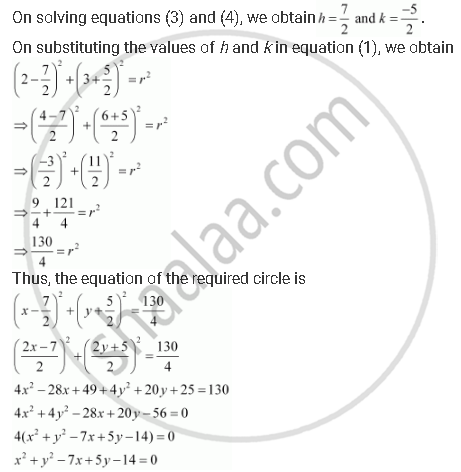CBSE (Arts) Class 11CBSE
Share
Notifications

View all notifications
Books Shortlist
Your shortlist is empty

# Find the Equation of the Circle Passing Through the Points (2, 3) and (–1, 1) and Whose Centre is on the Line X – 3y – 11 = 0. - CBSE (Arts) Class 11 - Mathematics

Login
Create free account

Forgot password?

#### Question

Find the equation of the circle passing through the points (2, 3) and (–1, 1) and whose centre is on the line – 3y – 11 = 0.

#### Solution

Let the equation of the required circle be (x – h)2 + (y – k)2 = r2.

Since the circle passes through points (2, 3) and (–1, 1),

(2 – h)2 + (3 – k)2 = r2 … (1)

(–1 – h)2 + (1 – k)2 = r2 … (2)

Since the centre (h, k) of the circle lies on line – 3y – 11 = 0,

– 3k = 11 … (3)

From equations (1) and (2), we obtain

(2 – h)+ (3 – k)2 = (–1 – h)2 + (1 – k)2

⇒ 4 – 4h + h2 + 9 – 6k + k2 = 1 + 2h + h2 + 1 – 2k + k2

⇒ 4 – 4h + 9 – 6k = 1 + 2h + 1 – 2k

⇒ 6h + 4k = 11 … (4)Is there an error in this question or solution?

#### APPEARS IN

Solution Find the Equation of the Circle Passing Through the Points (2, 3) and (–1, 1) and Whose Centre is on the Line X – 3y – 11 = 0. Concept: Concept of Circle.
S